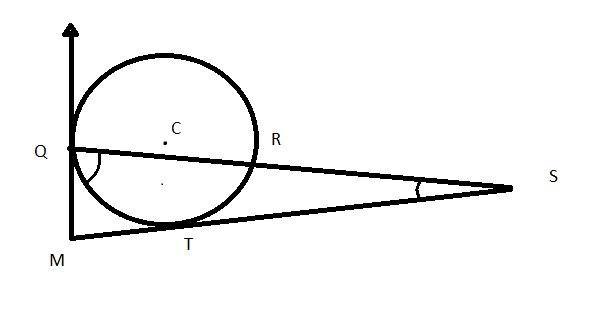# Can the Tangents help?

Geometry Level 3

In the diagram below, line segments $STM$ and $MQ$ are tangents to the circle at $T$ and $Q,$ respectively. $C$ is the center of the circle and does not lie on $QS.$ If $SRQ$ is a straight line, $SR=TR,$ and $\angle TSR=25^\circ,$ what is $\angle QMT$ in degrees?×

Problem Loading...

Note Loading...

Set Loading...